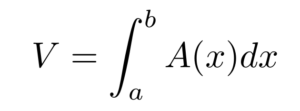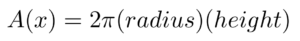# Cylindrical Shell Formula (The Shell Method)

Calculus Definitions > Cylindrical Shell Formula

The cylindrical shell method is a calculus-based strategy for finding the volume of a shape. The method is especially good for any shape that has radial symmetry, meaning that it always looks the same along a central axis. For things like flower vases, traffic cones, or wheels and axles, the cylindrical shell method is ideal.

## Deriving the Cylindrical Shell Formula

To begin, imagine that a three-dimensional object is divided into many thin slices with different areas, A. Integrate these areas together to find the total volume of the shape. This process is described by the general formula below:Where:

• V is the solid volume,
• a and b represent the edges of the solid, and
• A(x) is the area of each “slice.”

For the cylindrical shell method, these slices are hollow, thin cylinders, where the surface area of a cylinder is given byOne way to visualize the cylindrical shell approach is to think of a slice of onion. Each onion layer is skinny, but when it is wrapped in circular layers over and over again, it gives the onion substantial volume.

Substituting our cylindrical shell formula into the integral expression for volume from earlier, we haveTherefore, this formula represents the general approach to the cylindrical shell method.

## Example

Problem: Find the volume of a cone generated by revolving the function f(x) = x about the x-axis from 0 to 1 using the cylindrical shell method.

Solution

Step 1: Visualize the shape. A plot of the function in question reveals that it is a linear function.This simple linear function creates a cone when revolved around the x-axis, as shown below.With the cylindrical shell method, our strategy will be to integrate a series of infinitesimally thin shells.Step 2: Determine the area of the cylinder for arbitrary coordinates. For some point x between 0 and 1, the radius of the cylinder will be x, and the height will be 1-x. Therefore, the area of the cylindrical shell will beStep 3: Integrate the expression you got from Step 2 across the length of the shape to obtain the volume.Step 4: Verify that the expression obtained from volume makes sense in the question’s context.

The general formula for the volume of a cone is ⅓ π r2 h. So, V = ⅓ π (1)2 (1) = ⅓ π. So, our answer matches what we would expect for a cone.

In summary, any three-dimensional shape generated through revolution around a central axis can be analyzed using the cylindrical shell method, which involves these four simple steps.

CITE THIS AS:
Stephanie Glen. "Cylindrical Shell Formula (The Shell Method)" From StatisticsHowTo.com: Elementary Statistics for the rest of us! https://www.statisticshowto.com/cylindrical-shell-formula/